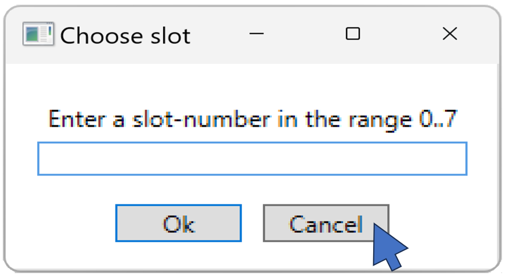C# provides many data types. For example double, int and string. A string variable can have the value null. This null value has a few pitfalls to be aware of. Value types like int and double cannot be null, but you can define nullable value types. This post explains that in more detail with simple examples. At the end it will be explained how to use if and else statements to execute different parts of the program.

In the previous article, we had written code that draws a hexagon. We’ll extend the code a little bit first, so that it draws a filled hexagon:

``````public static void WoopecMain()
{
var pen = Pen.CreateExample();
pen.IsDown = true;

var edgeLength = 50;
var numberOfEdges = 6;

pen.BeginFill();
for (var edgeCounter = 0; edgeCounter < numberOfEdges; edgeCounter++)
{
pen.Rotate(360.0 / numberOfEdges);
pen.Move(edgeLength);
}
pen.EndFill(Colors.Green);
}
``````

The outline that was drawn between the call of the method `BeginFill` and `EndFill` is filled with the color `Colors.Green`. Thus, a green filled hexagon is displayed on the screen.

The code always draws the hexagon in the center of the screen. In this article we want to extend the code further: The user should be able to specify where the hexagon is drawn.

### C# types

In the above program these three variables occur:

``````    var pen = Pen.CreateExample();
var edgeLength = 50.0;
var numberOfEdges = 6;
``````

These variables represent very different things:

• `pen` is an object of the Woopec graphics library. For example, we can call the `pen.Move(60)` method. However, we cannot execute `pen / 2`, for example.
• `edgeLength` is a decimal number. We can calculate `edgeLength / 2`, but we cannot call `edgeLength.Move(60)`, for example.
• `numberOfEdges` is a number without decimal places.

A variable is said to have a certain type. A variable in C# cannot change its type. It always remains the same. C# always knows exactly what type a variable is. That is, C# knows that `edgeLength` is a decimal number and therefore you must not call `edgeLength.Right(60)`. If you were to write that in the code, C# will generate an error message and the program will not be compiled.

In the examples above, C# automatically detects the type when compiling the program. We can therefore write a `var` in front of the variable. This way we tell C#: “If you can find out yourself what type the variable is, take this type”.

But we can also write the type explicitly:

``````    Pen pen = Pen.CreateExample();
double edgeLength = 50.0;
int numberOfEdges = 6;
``````

As long as it is crystal clear what type it is, we can stick to the simple notation with `var`. In all other cases we should write the type explicitly. For the C# compiler it would be no problem if we write `var`, but for the reader of the code it is better if we tell him explicitly what type it is. We will see an example of this in a moment.

### Query a text from the user

Suppose we want to ask the user what color our hexagon should have. We can use the `TextInput` method for this and assign the result to a variable:

``````    var color = pen.Screen.TextInput("Choose color", "Enter a color-name (for instance: red)");
``````

Calling the TextInput method displays a small dialog window where the user can enter a text: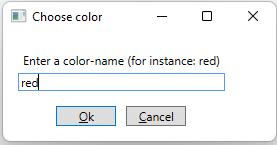The result of the input is assigned to the variable `color`. What type is the variable color? Is it really a color? Here it is better to write the type explicitly. The method TextInput returns a C# string and we write this type in front of the name of our variable:

``````    string colorName = pen.Screen.TextInput("Choose color", "Enter a color-name (for instance: red)");
``````

### C# string type

In programs you often have to deal with texts. That’s why the type ‘string’ is very important. You’d better have a look at some things about it on the internet now. For example the video The Basics of Strings , C# 101 in Microsoft Learn. For the Hexafour program we want to write here, we don’t need much more than what is described in this video. That’s why I won’t go much deeper into strings here.

### With null-strings there can be problems

We can use the value of variable colorName to fill our hexagon with this color:

``````    pen.EndFill(colorName);
``````

The hexagon is thus filled with the desired color. With one exception: What happens if the user clicked the Cancel button in the dialog window? If you start the program in the debugger (see this post) and click the Cancel button, the debugger shows this: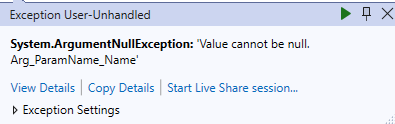The program somehow can’t handle the case when the user clicked the Cancel button. Restart the program again, put a breakpoint in the line with `pen.EndFill(colorName)`, and look at the value of the variable colorName (see previous post). The variable has the value null: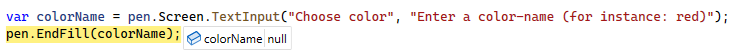The parameter of `pen.EndFill` must be a color, but the value null is not a color. The program cannot handle it and that is why an exception is thrown. We have to handle the case when a variable has the value null specially. We will see how to do this below. But first, let’s take a closer look at this strange null value. In the example above we asked the user for a text, if we ask for a number it gets even more interesting.

### Query a number from the user

In our planned game, the user should be able to specify in which “slot” she wants to place the next token. We mark the slots with consecutive numbers. The user should then be able to enter a number. There is a special woopec method for entering numbers:

``````    var userInput = pen.Screen.NumInput("Choose slot", "Enter a slot-number in the range 1..7", 4, 1, 7);
``````

We already know the first two parameters from the TextInput method. The third parameter is a default value. The fourth value specifies a minimum value for the input, and the fifth value a maximum value.

If the user enters a text that is not a number, or he enters a number that is not in the range of minimum and maximum value, the error is displayed and the user must correct the input: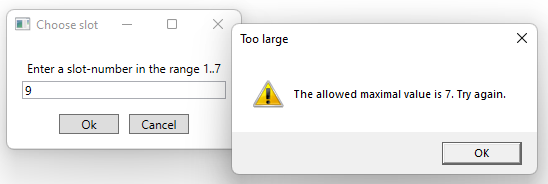So we can rely in our program code that the NumInput method returns an integer between 1 and 7. Except for one exception: if the user has selected the Cancel button, the value null is returned. And this is also a problem here:

### The value null is not the same as the value 0

Let’s change the program so that it adds the number 50 to `userInput`:

``````    var userInput = pen.Screen.NumInput("Choose slot", "Enter a slot-number in the range 1..7", 4, 1, 7);
var movement = userInput + 50;
``````

We run the program in the debugger and click the Cancel button in the number input. After that inputValue has the value null. Then we execute the line with the assignment to variable movement. What value does the variable movement have then? You might think the value 50. But this is not so, the value of movement is null. This shows that it makes a difference if the variable inputValue has the value null or the value 0. These are two completely different things. You cannot calculate with the value null.

### The nullable value type `int?` and how to make it an `int` again

The previous example becomes clearer if we do not use `var` but try to write the type of the variable explicitly. You might think that’s the way it is::

``````    int userInput = pen.Screen.NumInput("Choose slot", "Enter a slot-number in the range 1..7", 4, 1, 7);
``````

However, Visual Studio gives the following error for this line of code:

Cannot implicitly convert type ‘int?’ to ‘int’.

What does it mean? The NumInput method does not return a result of type int, but a result of type `int?` and this type does not match the type of the variable userInput. The type `int?` is a so-called nullable value type (see Microsoft documentation). The question mark after a variable of type `int?` indicates that such a variable may contain a number (i.e. an `int`) or the value null. On the contrary, a variable of type `int` may contain only a number and not null. Because the method NumInput returns a result of type `int?`, our variable userInput must also have this type:

``````    int? userInput = pen.Screen.NumInput("Choose slot", "Enter a slot-number in the range 1..7", 4, 1, 7);
``````

We cannot simply assign the value of variable userInput to another variable of type `int`. The following line of code is incorrect because userInput has a different type than movement:

``````    int movement = userInput;
``````

But this is how it goes:

``````    int movement = userInput.GetValueOrDefault();
``````

The `GetValueOrDefault()` method always returns a value of type int. In case of a real number it returns the number itself, and in case of null the number 0.

### Define C# conditions with `if`

In our program we must now somehow deal with the special case that the user has canceled the input. Here the `if` command of C# helps us. We can do it for example like this:

``````    var colorName = seymour.Screen.TextInput("Choose color", "Enter a color-name (for instance: red)");

if (colorName == null)
{
colorName = "red";
}
``````

The `if` command is as important as loops (see previous post). An `if` statement consists of the following parts:

• First comes the `if` keyword.
• Then comes the `if` condition. This must always be enclosed in round brackets. In our example the if-condition is `colorName == null`. The two characters `==` mean “is equal to”. That means in our example: The if-condition is fulfilled, if the value of variable colorName is equal to null.
• After that comes a code block with any number of lines. This code block starts and ends with curly brackets `{` and `}`. All lines of code between these two brackets belong to this code block and will be executed if the `if` condition is fulfilled.
• To make it easier to see where the code block starts and ends, the brackets are written on separate lines and the contents of the code block are indented. (We do this for `if` code blocks just as we do for loops).

### C# `if` und `else`

We can also tell the program what to do in the case when the if-condition is not true. This looks like this:

``````    var userInput = pen.Screen.NumInput("Choose slot", "Enter a slot-number in the range 1..3", 4, 1, 7);
if (userInput == null)
{
pen.Screen.Bye();
}
else
{
int slotNumber = userInput.GetValueOrDefault();
// ...
}
``````

The `else` command forms a unit with the previous if command. The code effectively says: If the userInput is `null` execute the first code block, else execute the second code block. In the above example:

• The `if` block is executed when variable userInput has the value null (because the user had clicked the Cancel button). In this case, the program is simply terminated. The `Bye()` Woopec method is used for this purpose.

• The `else` block is executed if variable userInput is not equal to null. We have to convert the value of userInput to an `int` type with `GetValueOrDefault`, otherwise we could not assign it to the variable slotNumber.

For more information on the `if` and `else` commands, see C# if statements and loops - conditional logic tutorial. You should have a look at this tutorial, because many things are explained there.

### All together in one program

If we put all the above pieces together, our program looks like this:

``````public static void WoopecMain()
{
var pen = Pen.CreateExample();

var edgeLength = 50;
var numberOfEdges = 6;

int? userInput = pen.Screen.NumInput("Choose slot", "Enter a slot-number in the range 1..7", 4, 1, 7);

if (userInput == null)
{
// The user want's to quit the game.
pen.Screen.Bye();
}
else
{
int slot = userInput.GetValueOrDefault();
pen.Move(slot * edgeLength);

pen.BeginFill();
for (var edgeCounter = 0; edgeCounter < numberOfEdges; edgeCounter++)
{
pen.Rotate(360.0 / numberOfEdges);
pen.Move(edgeLength);
}
pen.EndFill(Colors.Green);
}
}
``````

The program can already do a few things: It can ask the user for a slot and can draw a hexagon at the corresponding position depending on which slot the user has entered. For the full game we will have to write much more code. And the code is already starting to get messy again - it’s not really clean code anymore. We should change that soon, but first we have to get to know some more C# elements in the next post..

### Summary

This post is part of a series. You can find the previous post here and an overview here.

C#

Clean Code

• Use `var` only if it is crystal clear what type the variable is.

Woopec library

• Methods BeginFill and EndFill to draw a filled hexagon
• Methods TextInput and NumInput to query values from the user
• Method Bye to terminate the program